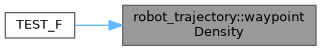moveit2 The MoveIt Motion Planning Framework for ROS 2.
robot_trajectory Namespace Reference

## Classes

class  RobotTrajectory
Maintain a sequence of waypoints and the time durations between these waypoints. More...

## Functions

MOVEIT_CLASS_FORWARD (RobotTrajectory)

std::ostream & operator<< (std::ostream &out, const RobotTrajectory &trajectory)
Operator overload for printing trajectory to a stream. More...

double pathLength (const RobotTrajectory &trajectory)
Calculate the path length of a given trajectory based on the accumulated robot state distances. The distance between two robot states is calculated based on the sum of active joint distances between the two states (L1 norm). More...

std::optional< double > smoothness (const RobotTrajectory &trajectory)
Calculate the smoothness of a given trajectory. More...

std::optional< double > waypointDensity (const RobotTrajectory &trajectory)
Calculate the waypoint density of a trajectory. More...

## ◆ MOVEIT_CLASS_FORWARD()

 robot_trajectory::MOVEIT_CLASS_FORWARD ( RobotTrajectory )

## ◆ operator<<()

 std::ostream & robot_trajectory::operator<< ( std::ostream & out, const RobotTrajectory & trajectory )

Operator overload for printing trajectory to a stream.

Definition at line 628 of file robot_trajectory.cpp.

Here is the call graph for this function: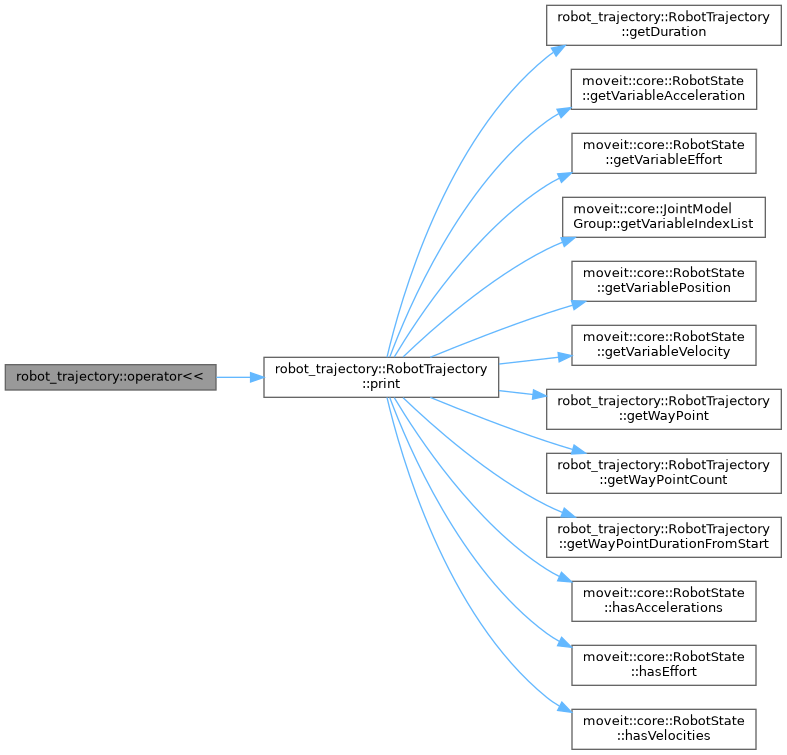## ◆ pathLength()

 double robot_trajectory::pathLength ( const RobotTrajectory & trajectory )

Calculate the path length of a given trajectory based on the accumulated robot state distances. The distance between two robot states is calculated based on the sum of active joint distances between the two states (L1 norm).

Parameters
 [in] trajectory Given robot trajectory
Returns
Length of the robot trajectory [rad]

Definition at line 634 of file robot_trajectory.cpp.

Here is the call graph for this function: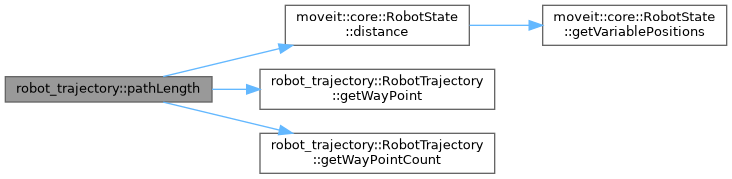Here is the caller graph for this function: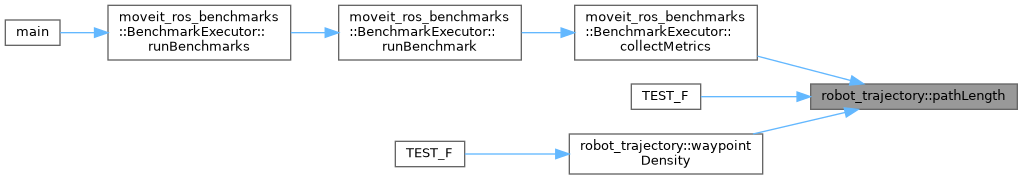## ◆ smoothness()

 std::optional< double > robot_trajectory::smoothness ( const RobotTrajectory & trajectory )

Calculate the smoothness of a given trajectory.

Parameters
 [in] trajectory Given robot trajectory
Returns
Smoothness of the given trajectory or nullopt if it is not possible to calculate the smoothness

(a + b);

(a + b);

Definition at line 646 of file robot_trajectory.cpp.

Here is the call graph for this function: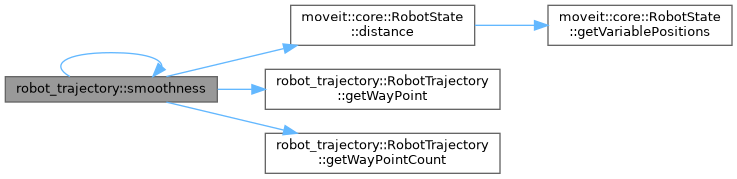Here is the caller graph for this function: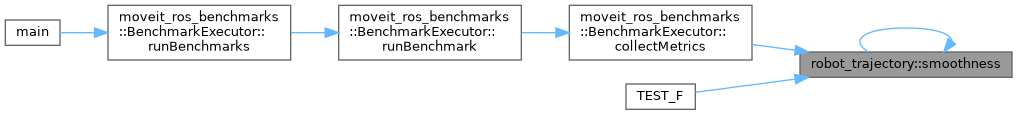## ◆ waypointDensity()

 std::optional< double > robot_trajectory::waypointDensity ( const RobotTrajectory & trajectory )

Calculate the waypoint density of a trajectory.

Parameters
 [in] trajectory Given robot trajectory
Returns
Waypoint density of the given trajectory or nullopt if it is not possible to calculate the density

Definition at line 684 of file robot_trajectory.cpp.

Here is the call graph for this function: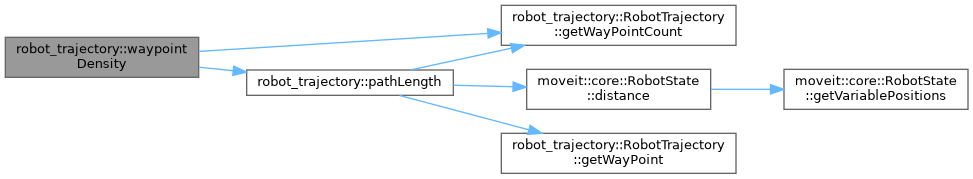Here is the caller graph for this function: